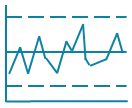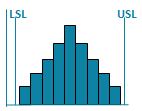# How to Use Excel for 1st, 2nd, 3rd Order Regression

## Use QI Macros Scatter Plot As a Starting Point

A QI Macros user recently called with what looked like a homework assignment. Normally, we don't help students with their homework, but I took a look anyway.

He wanted to know if QI Macros could do 2nd and 3rd order regression on his data.

You can do it in native Excel.### Here Is How to Calculate Second and Third Order Regression

1. Draw a scatter plot of the data. QI Macros scatter plot will automatically give you the first order linear equation. This gives us the first order answers: 2.39 and 2.86:As you can see, the trend line isn't a bad fit (R² = 0.9786), but we can investigate further.

2. Next, right click on the trend line and select Polynomial which gives us the second order answers (-0.22, 3.92, 0.82):This trend line is a better fit (R2=0.9961).

3. Next, change the Polynomial order to 3 and you get the third order answers (-0.066, 0.476, 1.82, 2.48):This trend line is a slightly better fit: (R2=0.9989).

Pretty simple, isn't it?

### Here's my point:

There's a lot of power hidden in Excel and I keep discovering more every day. Think outside the spreadsheet. What else can you do with Excel?

### Other Charts Included in QI Macros for Excel

Control ChartsHistogramsPareto ChartsBox PlotsFishbone Diagram